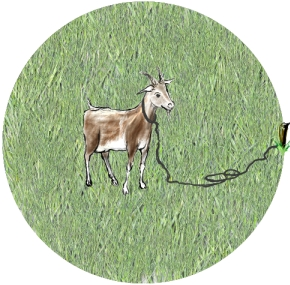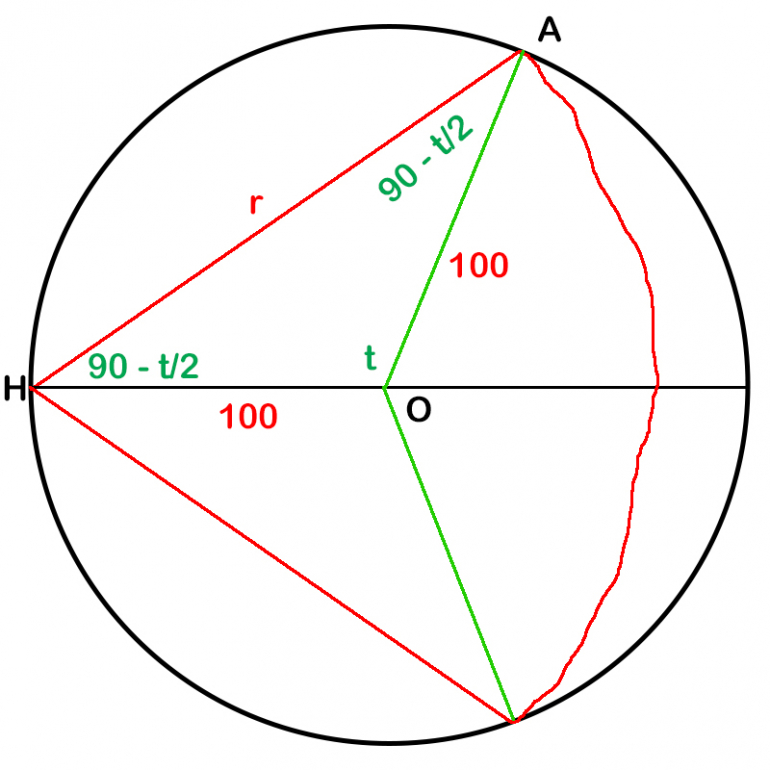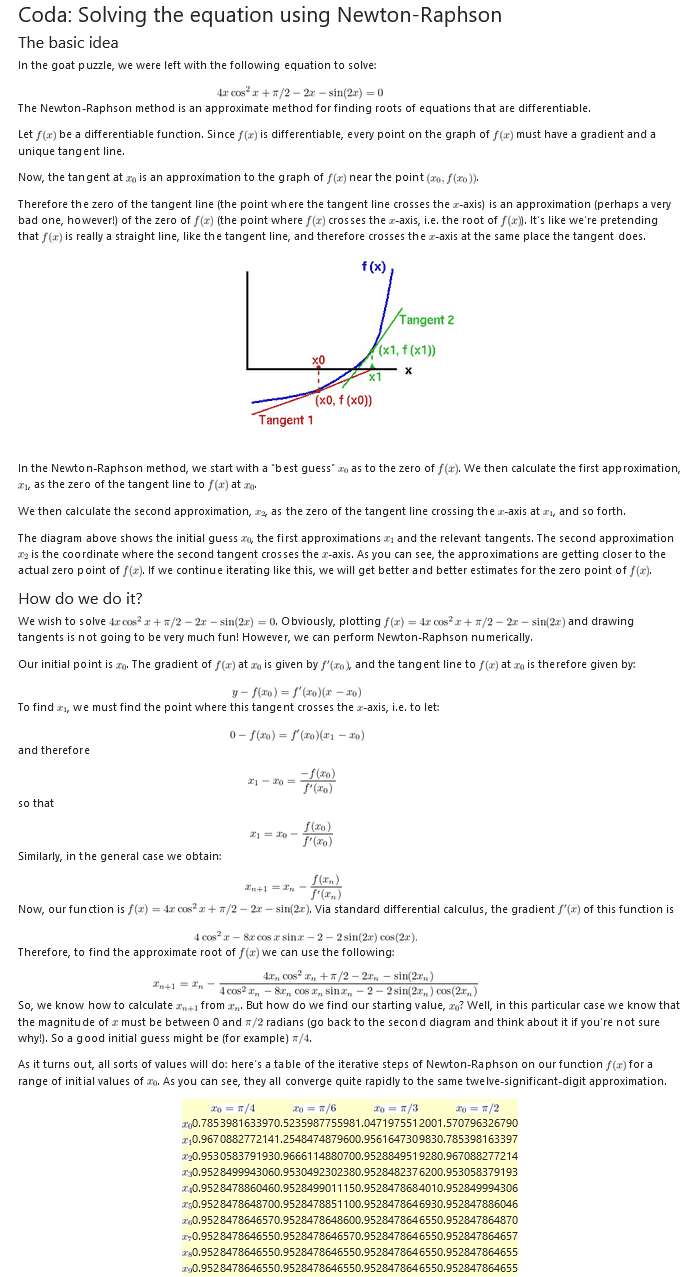## Poll

 I love math!20 votes (46.51%) Math is great.14 votes (32.55%) My religion is mathology.6 votes (13.95%) Women didn't speak to me until I was 30.2 votes (4.65%) Total eclipse reminder -- 04/08/202412 votes (27.9%) I steal cutlery from restaurants.3 votes (6.97%) I should just say what's on my mind.6 votes (13.95%) Who makes up these awful names for pandas?5 votes (11.62%) I like to touch my face.12 votes (27.9%) Pork chops and apple sauce.9 votes (20.93%)

43 members have voted

ThatDonGuyJoined: Jun 22, 2011
• Posts: 5680
March 27th, 2022 at 4:02:09 PM permalink
After some serious number crunching (doing a brute force computing search), I get the following probabilities for each number of lines:

0 lines: 20,399,310,508,737,822,613,691,645 / 54,208,514,626,416,667,373,209,017 (1 / 2.657)
1 line: 453,138,748,079,790,278,790,802,600 / 1,246,795,836,407,583,349,583,807,391 (1 / 2.751)
2 lines: 75,184,281,240,179,546,107,280,550 / 415,598,612,135,861,116,527,935,797 (1 / 5.528)
3 lines: 74,961,880,766,219,254,839,487,700 / 1,246,795,836,407,583,349,583,807,391 (1 / 16.63)
4 lines: 19,111,256,934,190,578,133,923,260 / 1,246,795,836,407,583,349,583,807,391 (1 / 65.24)
5 lines: 22,485,961,213,854,457,920,528 / 7,044,044,273,489,171,466,575,183 (1 / 313.26)
6 lines: 4,112,990,939,009,607,535,740 / 7,044,044,273,489,171,466,575,183 (1 / 1712.6)
7 lines: 12,492,941,476,251,446,600 / 132,906,495,726,210,782,388,211 (1 / 10,639)
8 lines: 41,224,371,704,971,650 / 2,712,377,463,800,220,048,739 (1 / 65,795)
9 lines: 104,423,327,361,600 / 57,710,158,804,260,001,037 (1 / 552,656)
10 lines: 1,071,008,485,760 / 2,509,137,339,315,652,219 (1 / 2,342,780)
11 lines: 0
12 lines: 33,469,015,180 / 2,509,137,339,315,652,219 (1 / 74,968,962)
GialmereJoined: Nov 26, 2018
• Posts: 2589
March 28th, 2022 at 8:07:06 AM permalink
Here's a classic puzzle that's harder than it looks...A grassy field is in the shape of a circle of radius 100m and is enclosed by a circular fence.

A goat is attached by a rope to a hook, at a fixed point on the fence.

To stop the goat getting too fat, the farmer wants to make sure that it can only reach half of the grass in the field.

How long should the rope be?
Have you tried 22 tonight? I said 22.
ThatDonGuyJoined: Jun 22, 2011
• Posts: 5680
March 28th, 2022 at 10:32:49 AM permalink

Is there an "exact" value for this? I can come up with a solution, but it involves a bit of trigonometry in the answer.

GialmereJoined: Nov 26, 2018
• Posts: 2589
March 28th, 2022 at 10:39:24 AM permalink
Quote: ThatDonGuy

Is there an "exact" value for this? I can come up with a solution, but it involves a bit of trigonometry in the answer.

An approximation rounding the rope to the nearest meter in length is acceptable.
Have you tried 22 tonight? I said 22.
Ace2Joined: Oct 2, 2017
• Posts: 2318
March 28th, 2022 at 10:55:19 AM permalink
The weather channel app on my phone listed a 100% chance of rain this morning but it never did rain...now the sky is clearing and there is no more forecast for rain today

What are the odds of something with a 100% chance not occurring?
It�s all about making that GTA
ThatDonGuyJoined: Jun 22, 2011
• Posts: 5680
Thanks for this post from:March 28th, 2022 at 11:07:21 AM permalink
Quote: Gialmere

Quote: ThatDonGuy

Is there an "exact" value for this? I can come up with a solution, but it involves a bit of trigonometry in the answer.

An approximation rounding the rope to the nearest meter in length is acceptable.Sorry about that red arc on the right; you would think Adobe Photoshop Elements would include an easy way to draw an arc.

Let O be the center of the field, H be the point where the rope is hooked to the fence,
and A be one of the two points on the fence that is the length of the rope away from H.
Let r be the length of the rope; HA = r.
The field is symmetric with respect to the diameter that includes H, so only half of the value will be calculated, and the result multiplied by 2 to obtain the final result.

One formula for the area of a triangle = 1/2 the product of the lengths of two sides * the sine of the angle where they meet
The area covered in half of the field = PI r^2 * (90 - t/2) / 360 + PI * 100^2 * t / 360 - 1/2 * 100^2 sin t
Bisect AH; sin (t / 2) = (r / 2) / 100 = r / 200
t = 2 arcsin (r / 200)

The total area covered = 2 (PI r^2 * (90 - t/2) / 360 + PI * 100^2 * t / 360 - 1/2 * 100^2 sin t)
= 2 (PI r^2 * (90 - arcsin(r / 200)) / 360 + PI * 500 arcsin(r / 200) / 9 - 5000 sin t)
= PI r^2 * (90 - arcsin(r / 200)) / 180 + PI * 1000 arcsin(r / 200) / 9 - 10,000 sin t

Since this equals half of the area of the field, which is 5000 PI:

5000 PI = PI r^2 * (90 - arcsin(r / 200)) / 180 + PI * 1000 arcsin(r / 200) / 9 - 10,000 sin t
5000 = r^2 * (90 - arcsin(r / 200)) / 180 + 1000 arcsin(r / 200) / 9 - 10,000 / PI * sin t

sin t = sin (2 * t/2) = 2 sin (t/2) cos (t/2) = 2 * r / 200 * sqrt(1 - r^2 / 40,000) = r sqrt(1 - r^2 / 40,000) / 100

5000 = r^2 * (90 - arcsin(r / 200)) / 180 + 1000 arcsin(r / 200) / 9 - 100 / PI * r sqrt(1 - r^2 / 40,000)

The length of the rope = about 116 m

acesideJoined: May 14, 2021
• Posts: 326
March 28th, 2022 at 12:06:20 PM permalink
This can be solved by using polar equations.

The polar equation of the larger circle is
r=x.

The polar equation of the smaller circle is
r=200 cos(theta).

Solving the set of two equations to find the intersection points, and thus the limits of integration.

Using the polar equations and the intersection points to do an area integration, we should find it.
ThatDonGuyJoined: Jun 22, 2011
• Posts: 5680
March 28th, 2022 at 12:31:48 PM permalink
Quote: aceside

This can be solved by using polar equations.

The polar equation of the larger circle is
r=x.

The polar equation of the smaller circle is
r=200 cos(theta).

Solving the set of two equations to find the intersection points, and thus the limits of integration.

Using the polar equations and the intersection points to do an area integration, we should find it.

But doesn't the range of the integration depend on the length of the rope?
unJonJoined: Jul 1, 2018
• Posts: 4067
March 28th, 2022 at 12:35:01 PM permalink
Quote: ThatDonGuy

Quote: Gialmere

Quote: ThatDonGuy

Is there an "exact" value for this? I can come up with a solution, but it involves a bit of trigonometry in the answer.

An approximation rounding the rope to the nearest meter in length is acceptable.Sorry about that red arc on the right; you would think Adobe Photoshop Elements would include an easy way to draw an arc.

Let O be the center of the field, H be the point where the rope is hooked to the fence,
and A be one of the two points on the fence that is the length of the rope away from H.
Let r be the length of the rope; HA = r.
The field is symmetric with respect to the diameter that includes H, so only half of the value will be calculated, and the result multiplied by 2 to obtain the final result.

One formula for the area of a triangle = 1/2 the product of the lengths of two sides * the sine of the angle where they meet
The area covered in half of the field = PI r^2 * (90 - t/2) / 360 + PI * 100^2 * t / 360 - 1/2 * 100^2 sin t
Bisect AH; sin (t / 2) = (r / 2) / 100 = r / 200
t = 2 arcsin (r / 200)

The total area covered = 2 (PI r^2 * (90 - t/2) / 360 + PI * 100^2 * t / 360 - 1/2 * 100^2 sin t)
= 2 (PI r^2 * (90 - arcsin(r / 200)) / 360 + PI * 500 arcsin(r / 200) / 9 - 5000 sin t)
= PI r^2 * (90 - arcsin(r / 200)) / 180 + PI * 1000 arcsin(r / 200) / 9 - 10,000 sin t

Since this equals half of the area of the field, which is 5000 PI:

5000 PI = PI r^2 * (90 - arcsin(r / 200)) / 180 + PI * 1000 arcsin(r / 200) / 9 - 10,000 sin t
5000 = r^2 * (90 - arcsin(r / 200)) / 180 + 1000 arcsin(r / 200) / 9 - 10,000 / PI * sin t

sin t = sin (2 * t/2) = 2 sin (t/2) cos (t/2) = 2 * r / 200 * sqrt(1 - r^2 / 40,000) = r sqrt(1 - r^2 / 40,000) / 100

5000 = r^2 * (90 - arcsin(r / 200)) / 180 + 1000 arcsin(r / 200) / 9 - 100 / PI * r sqrt(1 - r^2 / 40,000)

The length of the rope = about 116 m

Your drawing is funny. Note that the rope should bisect the circle at points that are to the left of the center of the circle, not the right of the center of the circle.
The race is not always to the swift, nor the battle to the strong; but that is the way to bet.
GialmereJoined: Nov 26, 2018
• Posts: 2589
March 28th, 2022 at 4:45:51 PM permalink
Quote: ThatDonGuySorry about that red arc on the right; you would think Adobe Photoshop Elements would include an easy way to draw an arc.

Let O be the center of the field, H be the point where the rope is hooked to the fence,
and A be one of the two points on the fence that is the length of the rope away from H.
Let r be the length of the rope; HA = r.
The field is symmetric with respect to the diameter that includes H, so only half of the value will be calculated, and the result multiplied by 2 to obtain the final result.

One formula for the area of a triangle = 1/2 the product of the lengths of two sides * the sine of the angle where they meet
The area covered in half of the field = PI r^2 * (90 - t/2) / 360 + PI * 100^2 * t / 360 - 1/2 * 100^2 sin t
Bisect AH; sin (t / 2) = (r / 2) / 100 = r / 200
t = 2 arcsin (r / 200)

The total area covered = 2 (PI r^2 * (90 - t/2) / 360 + PI * 100^2 * t / 360 - 1/2 * 100^2 sin t)
= 2 (PI r^2 * (90 - arcsin(r / 200)) / 360 + PI * 500 arcsin(r / 200) / 9 - 5000 sin t)
= PI r^2 * (90 - arcsin(r / 200)) / 180 + PI * 1000 arcsin(r / 200) / 9 - 10,000 sin t

Since this equals half of the area of the field, which is 5000 PI:

5000 PI = PI r^2 * (90 - arcsin(r / 200)) / 180 + PI * 1000 arcsin(r / 200) / 9 - 10,000 sin t
5000 = r^2 * (90 - arcsin(r / 200)) / 180 + 1000 arcsin(r / 200) / 9 - 10,000 / PI * sin t

sin t = sin (2 * t/2) = 2 sin (t/2) cos (t/2) = 2 * r / 200 * sqrt(1 - r^2 / 40,000) = r sqrt(1 - r^2 / 40,000) / 100

5000 = r^2 * (90 - arcsin(r / 200)) / 180 + 1000 arcsin(r / 200) / 9 - 100 / PI * r sqrt(1 - r^2 / 40,000)

The length of the rope = about 116 m

Correct!!

Well done.-------------------------------------------------------------------------Have you tried 22 tonight? I said 22.